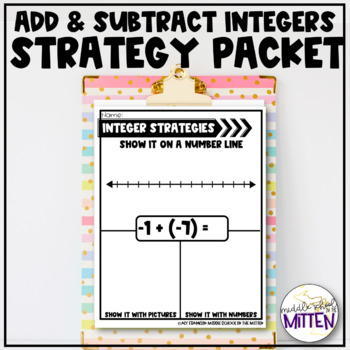# Adding and Subtracting Integers Strategies PacketSubject
Resource Type
File Type

PDF

(462 KB|30 pages)
Product Rating
Standards
• Product Description
• StandardsNEW

This packet is a complete resource for practice using three strategies for teaching the basics of adding and subtracting positive and negative numbers. Students solve each problem in three ways; using positive/negative symbols or pictures, number lines, and with numbers. Through solving each problem in multiple ways, students build connections between techniques and build a deeper understanding. This resource currently includes a total of 28 problems, as well as three numberless templates for unlimited problems. My middle school students found these helpful in truly understanding what adding and subtracting integers meant and looked like.

Please view the preview for more details.

Apply properties of operations as strategies to add and subtract rational numbers.
Understand subtraction of rational numbers as adding the additive inverse, 𝘱 – 𝘲 = 𝘱 + (–𝘲). Show that the distance between two rational numbers on the number line is the absolute value of their difference, and apply this principle in real-world contexts.
Understand 𝘱 + 𝘲 as the number located a distance |𝘲| from 𝘱, in the positive or negative direction depending on whether 𝘲 is positive or negative. Show that a number and its opposite have a sum of 0 (are additive inverses). Interpret sums of rational numbers by describing real-world contexts.
Describe situations in which opposite quantities combine to make 0. For example, a hydrogen atom has 0 charge because its two constituents are oppositely charged.
Apply and extend previous understandings of addition and subtraction to add and subtract rational numbers; represent addition and subtraction on a horizontal or vertical number line diagram.
Total Pages
30 pages
Included
Teaching Duration
N/A
Report this Resource to TpT
Reported resources will be reviewed by our team. Report this resource to let us know if this resource violates TpT’s content guidelines.